# Trigonometric Functions Graph of Sin𝛉 and Cos𝛉 using GeoGebra

Trigonometry is the branch of mathematics that deals with the relationship between ratios of the sides of a right-angled triangle with its angles.

Trigonometric Functions

There are six basic trigonometric functions used in Trigonometry. These functions are trigonometric ratios. The six basic trigonometric functions are sine function, cosine function, secant function, co-secant function, tangent function, and co-tangent function. The trigonometric functions and identities are the ratio of sides of a right-angled triangle. The sides of a right triangle are the perpendicular side, hypotenuse, and base, which are used to calculate the sine, cosine, tangent, secant, cosecant, and cotangent values using trigonometric formulas.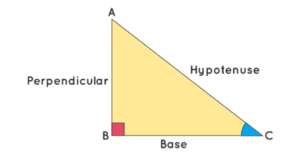Unit Circle and Trigonometric Values

Unit circle can be used to calculate the values of basic trigonometric functions- sine, cosine, and tangent. The following diagram shows how trigonometric ratios sine and cosine can be represented in a unit circle.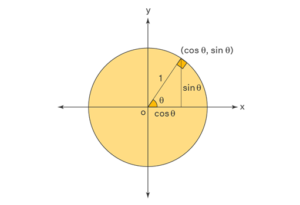Trigonometric Functions Graph

The graphs of trigonometric functions have the domain value of θ represented on the horizontal x-axis and the range value represented along the vertical y-axis. The graph of Sinθ passes through the origin and the graph of Cosθ does not pass through the origin. The range of Sinθ and Cosθ is limited to [-1, 1].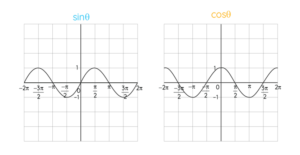Let’s see the practical execution of the trigonometric function graphs of Sinθ and Cosθ using GeoGebra.

Steps for trigonometric function graphs of Sinθ:

• Plot two points A and B and draw a unit circle (radius=1cm).
• Mark a point C on the circle and measure angle BAC. Rename the angle as a.
• Change the settings of the x-axis by giving the distance as π/2.
• Draw a line segment between points A and C.
• Now give the input as Segment (C, (x(C),0)).
• A line drawn from C to the x-axis and point C changes with the angle change can be seen.
• Now click on settings and change the line style and colour.
• Now give input as f(x)=sin(x),0<=x<=a.
• A sine wave has drawn as per the given range can be seen.
• Now plot a point on the curve end D.
• Now, to see the change between 0 degrees to 360 degrees, give the input a     Dynamic coordinate (D, a, y(C)).
• We can see the graph is drawn for every change in angle of BAC and a sine function graph simultaneously.
• Random point E appears along with the curve.
• Now give the input as Segment (E, (x(E),0)).
• Now click on settings and change the line style and colour.
• By clicking on the animation icon, the desired output can be visualized.

The figure shows the sine wave obtained using GeoGebra. It can be observed that the graph of Sinθ passes through the origin.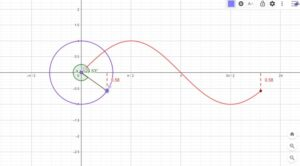https://www.geogebra.org/classic/cdkf3rme

Steps for trigonometric function graph of Cosθ:

• Plot two points A and B and draw a unit circle (radius=1cm).
• Mark a point C on the circle and measure angle BAC. Rename the angle as a.
• Change the settings of the x-axis by giving the distance as π/2.
• Draw a line segment between points A and C.
• Now give the input as Segment (C, (x(C),0)).
• A line drawn from C to the x-axis and point C changes with the angle change can be seen.
• Now click on settings and change the line style and colour.
• Now give input as f(x)=Cos(x),0<=x<=a.
• A sine wave has drawn as per the given range can be seen.
• Now plot a point on the curve end D.
• Now, to see the change between 0 degrees to 360 degrees, give the input a Dynamic coordinate (D, a, y(C)).
• We can see the graph is drawn for every change in angle of BAC and a sine function graph simultaneously.
• Random point E appears along with the curve.
• Now give the input as Segment (E, (x(E),0)).
• Now click on settings and change the line style and colour.
• By clicking on the animation icon, the desired output can be visualized.

The figure shows the Cosine wave obtained using GeoGebra. It can be observed that the graph of Cosθ does not pass through the origin.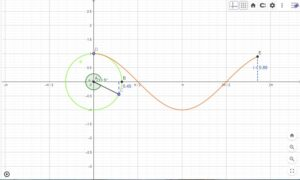https://www.geogebra.org/classic/bcqtxcjr